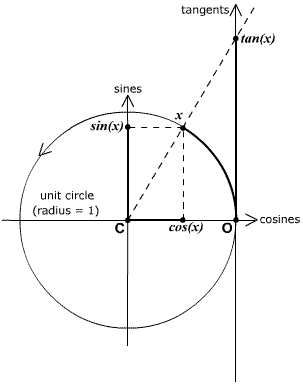## MAT125 Spring 2011 Review for Midterm I

1.1 Understand the definition of function (box, p.12) and the importance of the word "exactly." The Vertical Line Test (p.17) shows exactly what "exactly" means in terms of graphs (Exercises 5-8). Be able to ascertain the domain of a function either from the formula (Example 6, Exercises 29-33) or the graph (Example 1,Exercises 1, 2, 5-8). Practice sketching graphs (Exercises 36-39), especially for piecewise-defined functions (Exercises 43-46).

1.2 Be familiar with the "essential functions." Linear (Example 1, Exercises 10,11,15,18); be able to interpret slope and y-intercept. Polynomial: know what the degree is (p.29), (Example 4, Exercise 3). Power functions: understand that the 1/n power (n a positive integer) corresponds to the nth root; and that if n is even, f(x) = x1/n is only defined for positive x; understand negative powers as reciprocals of the positive: x-n = 1/xn; be comfortable with the law of exponents in this context: xaxb = xa+b,   xa/xb = xa-b,   (xa)b = xab; a and b can be integral, fractional, positive, negative, anything.Trigonometric functions: keep this diagram firmly and permanently in mind to understand sine, cosine and tangent as functions of x in radians. Starting at O (the right-hand intersection of the unit circle with the horizontal axis), go a distance x counterclockwise along the circle. Projection onto the horizontal (cosines) axis then gives cos(x); projection onto the vertical (sines) axis gives sin(x); the line through C (center of the circle) and x intersects the tangents axis at tan(x). (The tangents axis is tangent to the circle at O.)
Elementary properties of sin, cos, tan can be retrieved from this diagram, e.g. -1 ≤ sin(x) ≤ 1,   -1 ≤ cos(x) ≤ 1,   tan(x) = sin(x)/cos(x) (similar triangles),   sin2(x) + cos2(x) = 1 (Pythagorean theorem),   sin(x + 2π) = sin(x),   cos(x + 2π) = cos(x) (2π is length of circle), etc.

Exponential and logarithmic functions, also "essential," are covered in § 1.5.

1.3 Understand how translations work (Box, p.38 and Figure 1); remember that f(x-c) has the graph of f(x) shifted c units to the right, and understand why! (Exercise 3ade). Same for stretching and reflecting (Box, p.39 and Figure 2); remember that for example f(2x) has the graph of f(x) compressed by a factor of 2, and understand why. Also understand why -f(x) and f(-x) do very different things to f(x). Exercises 1,2,3,4,5.
Understand that when two functions are combined by +, x, - and / the domain of the combination is the intersection of the two domains: the set of points where both functions are defined, plus no zeroes in the denominator! Exercises 29,30. Be able to "complete the square" (Example 2, Exercise 12). Remember that in the composition f(g(x)) the function g is applied first. (Example 6, Exercises 31-36).

1.5 Be able to sketch the graph of an exponential function f(x) = ax: f(0)= 1 always. The function increases from 0 to infinity if a > 1, it's constant and equal to 1 if a = 1, it decreases from infinity to 0 if 0 < a < 1. Only positive a are considered! (Exercises 7,8,9). Be familiar and completely comfortable with the Laws of Exponents (box, p.54). Know what e is (p.57). (Example 4, Exercises 14,15,16).

1.6 Understand that a function can only have an inverse if it is one-one, i.e. it satisfies the Horizontal Line Test (box, p.61). Examples 1,2 are fundamental. Exercises 5-8. Understand and be able to apply the algorithm (box, p.64) to calculate the inverse of a one-one function. (Example 4, Exercises 21,22).
In particular the exponential function f(x) = ax for any a not equal to 1 has an inverse, called "logarithm to the base a," and written loga. Understand and be comfortable with the equivalences between logax = y and x = ay, etc. (Boxes on p.65!!). Be comfortable with the Laws of Logarithms (Box, p.65). (Example 6, Exercises 35,36). Know the natural logarithm ln x = logex, and know the change-of-base formula logax = (ln x)/(ln a) (Box, p.67 Example 10 -requires a calculator).

2.1 Be able to sketch secant lines through a point on a curve, and the tangent line to the curve at that point, and understand how to use slopes of secants to estimate the slope of the tangent (Examples 1,2,Exercises 3, 4 -require calculator). Be able to calculate average velocities from distance-time data and to use them to estimate instantaneous velocity (Exercises 6.7).

2.2 Understand that the limit of a function f at a does not involve the value f(a) (which may not even be defined). (Definition, p.95). (Example 1). Also definitions of left- and right-hand limits (Box, p.100 and below). Be able to estimate limx->af(x) as well as one-sided limits from inspection of the graph of f (Exercises 3,4,5,6,12).

Use the Chapter Reviews for further reviewing.

Chapter 1

• Concept Check 1 through 12.
• True-False 1 through 11.
• Exercises 1 through 16, 18,19,20.
Chapter 2
• Concept Check 1, 2, 3, 4
• True-False 1 through 8.
• Exercises 1 through 18

Feb 16 2011